Most Affordable JEE | NEET | 8,9,10 Preparation by Kota's Top IITian Doctor Faculties

# NCERT Solutions For Class 12 Maths Chapter 10 Miscellaneous Exercise - Vector Algebra - Free PDF Download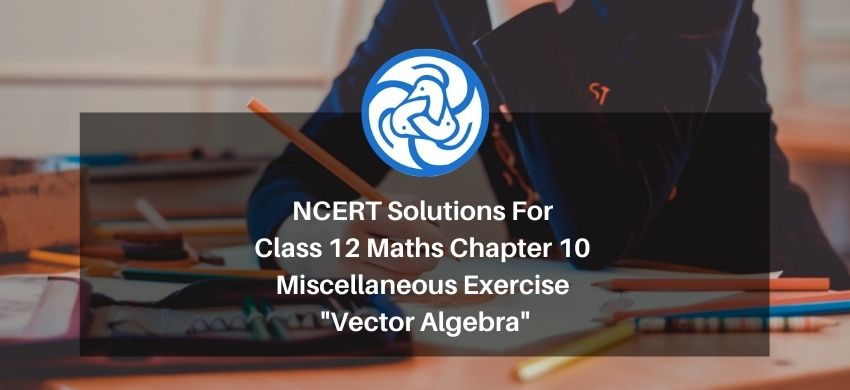`
Hey, are you a class 12 Student and Looking for Ways to Download NCERT Solutions For Class 12 Maths Chapter 10 Miscellaneous Exercise? If Yes then you are at the right place.

All the solutions of Class 12 Maths Chapter 10 Miscellaneous Exercise in PDF is prepared by Kota’s top IITian’s Faculties by keeping Simplicity in mind.

If you want to score high in your class 12 Maths Exam then it is very important for you to have a good knowledge of all the important topics, so to learn and practice those topics you can use eSaral NCERT Solutions.

So, without wasting more time Let’s start.

### Download The PDF of NCERT Solutions For Class 12 Math Chapter 10 Miscellaneous Exercise "Vector Algebra"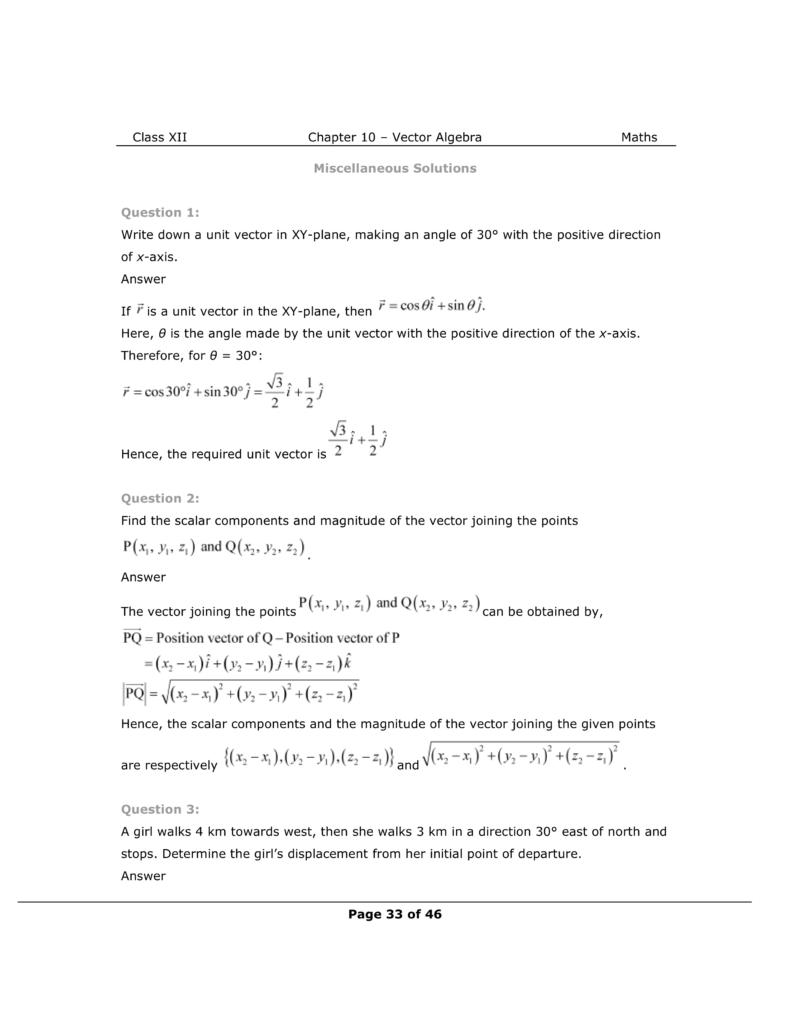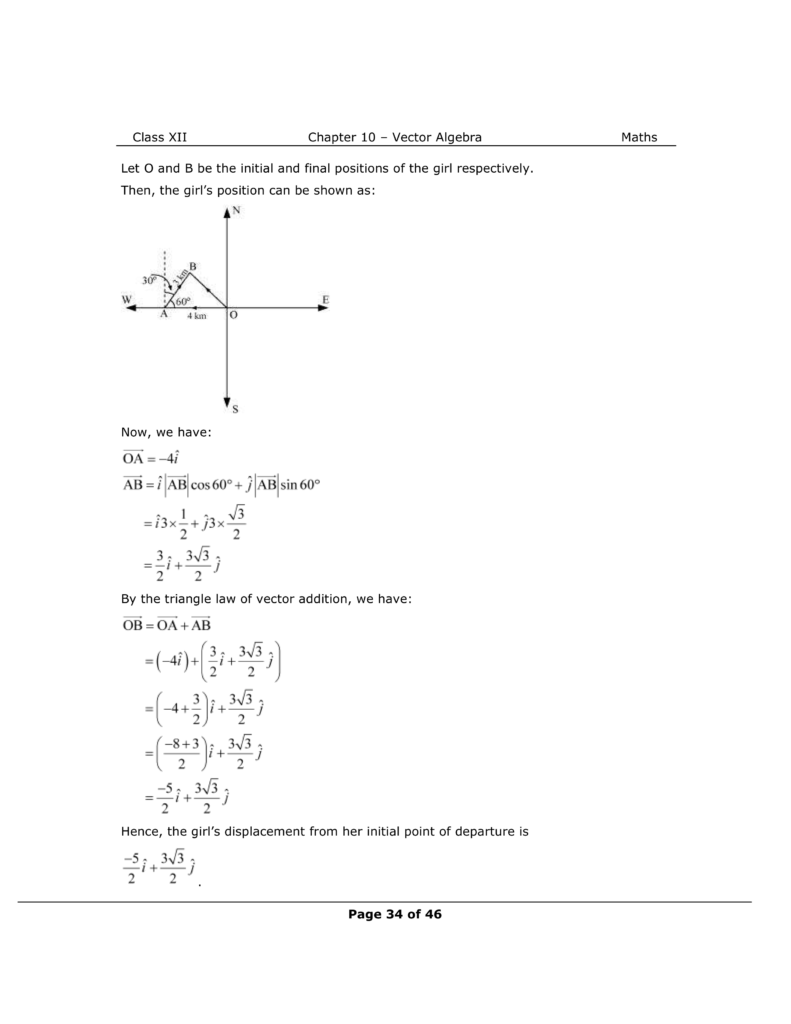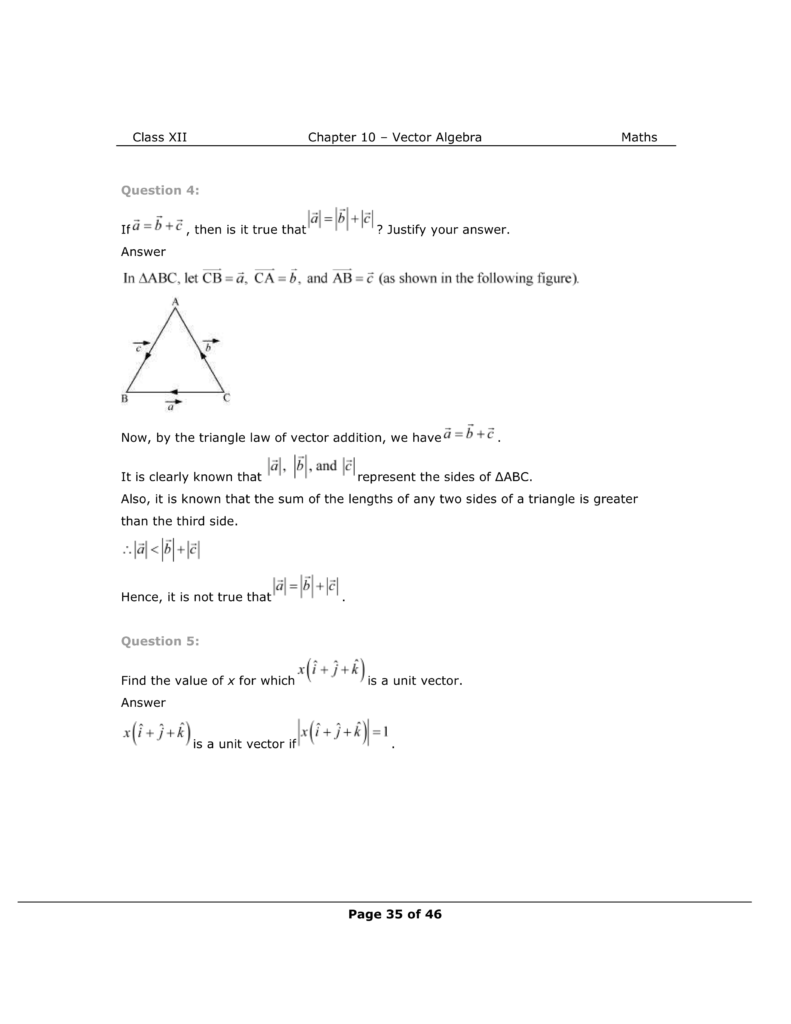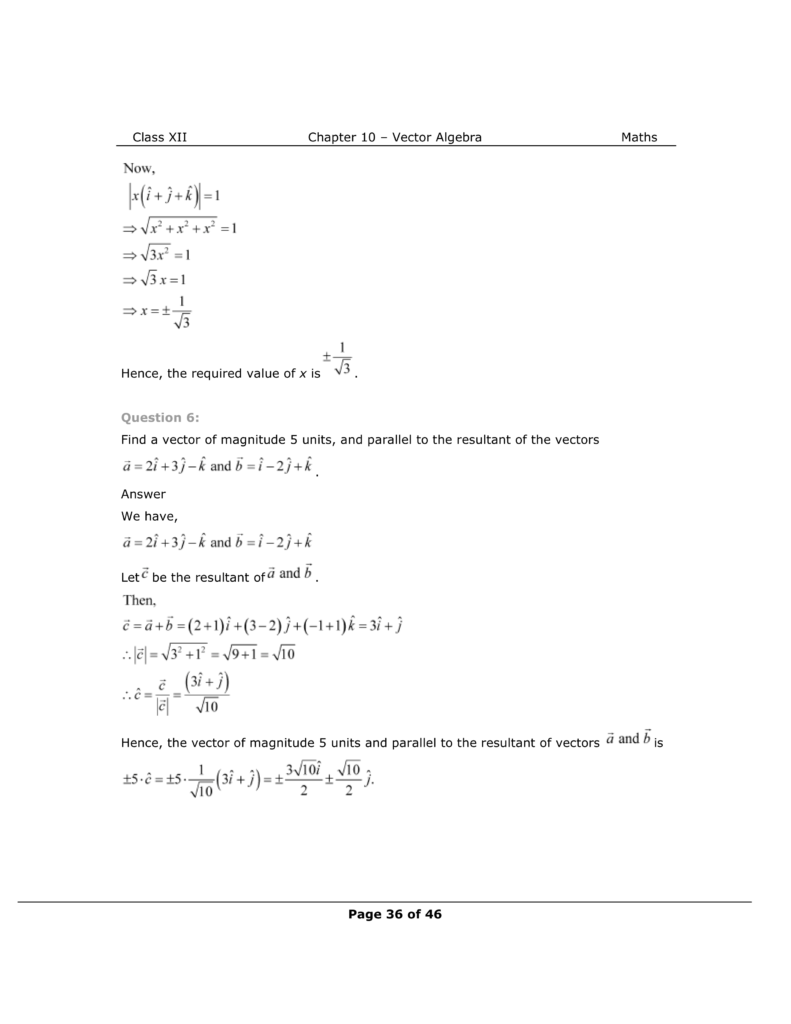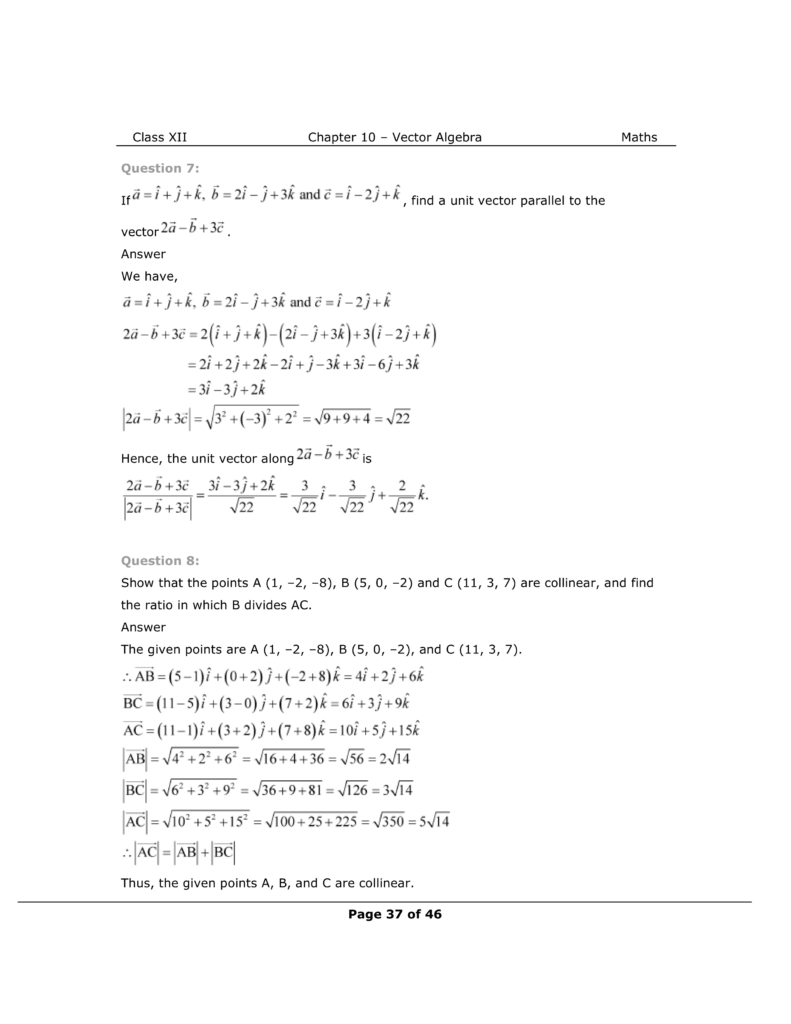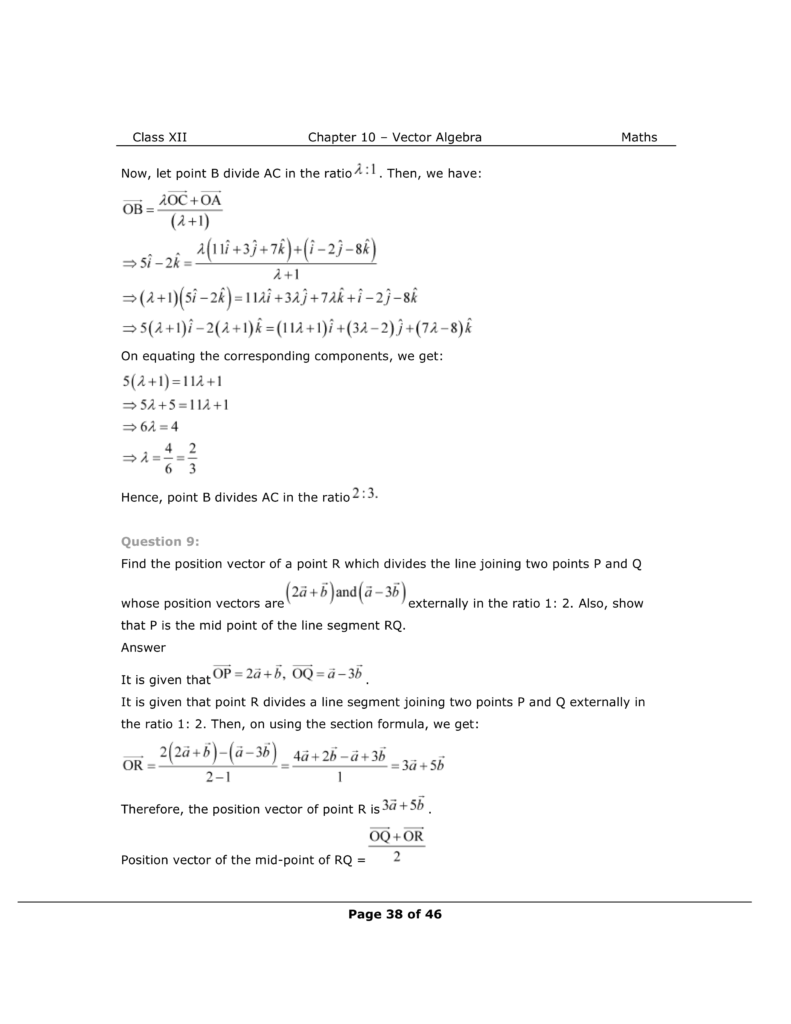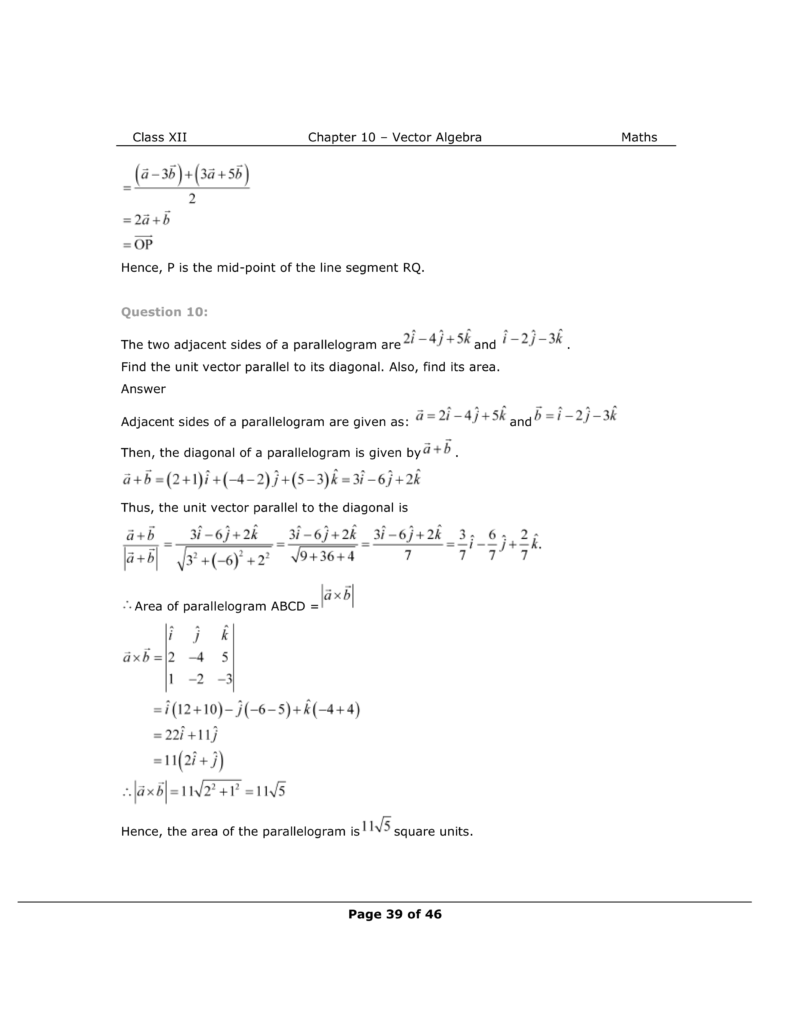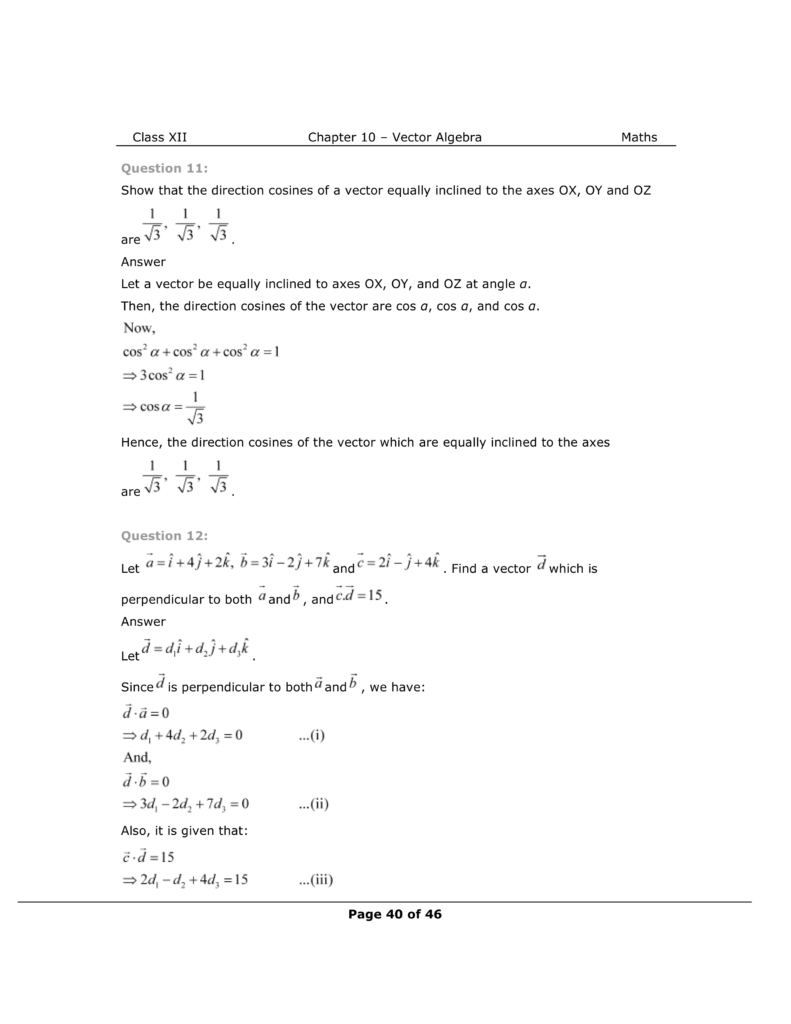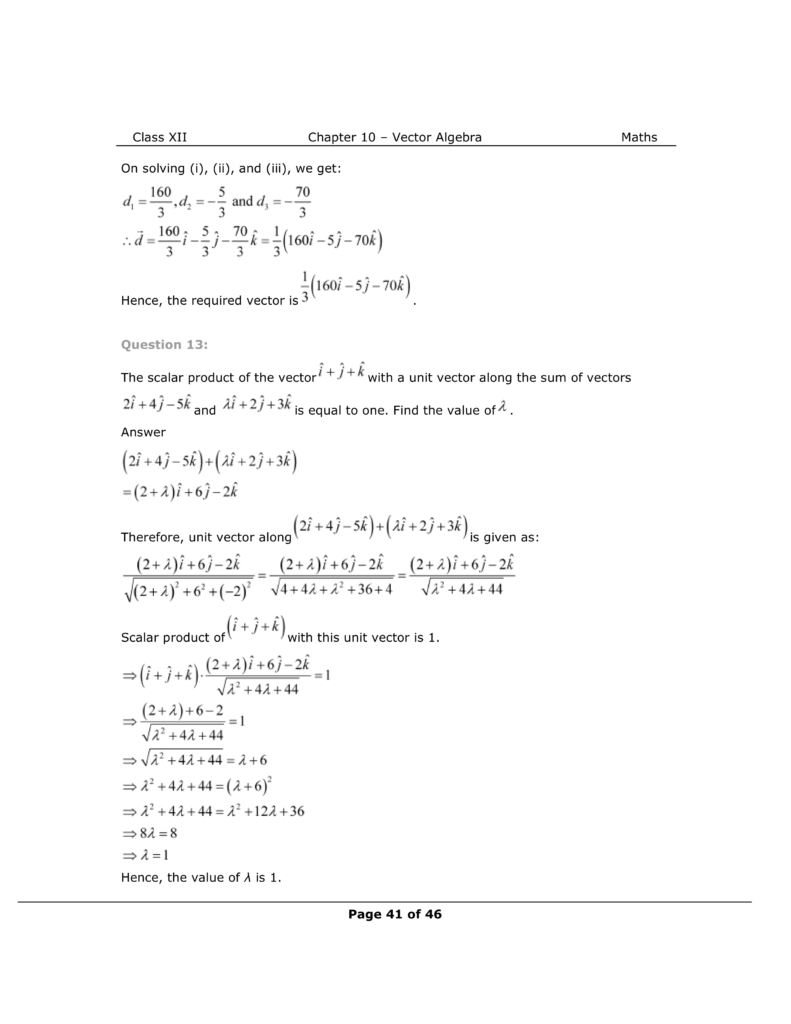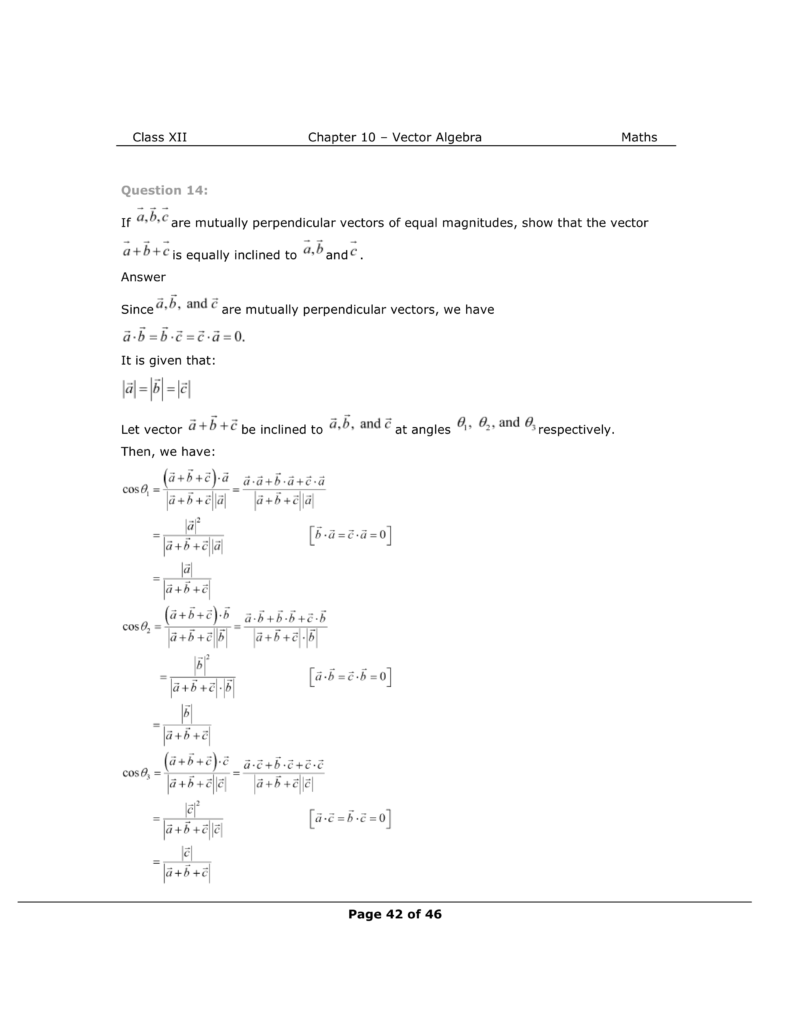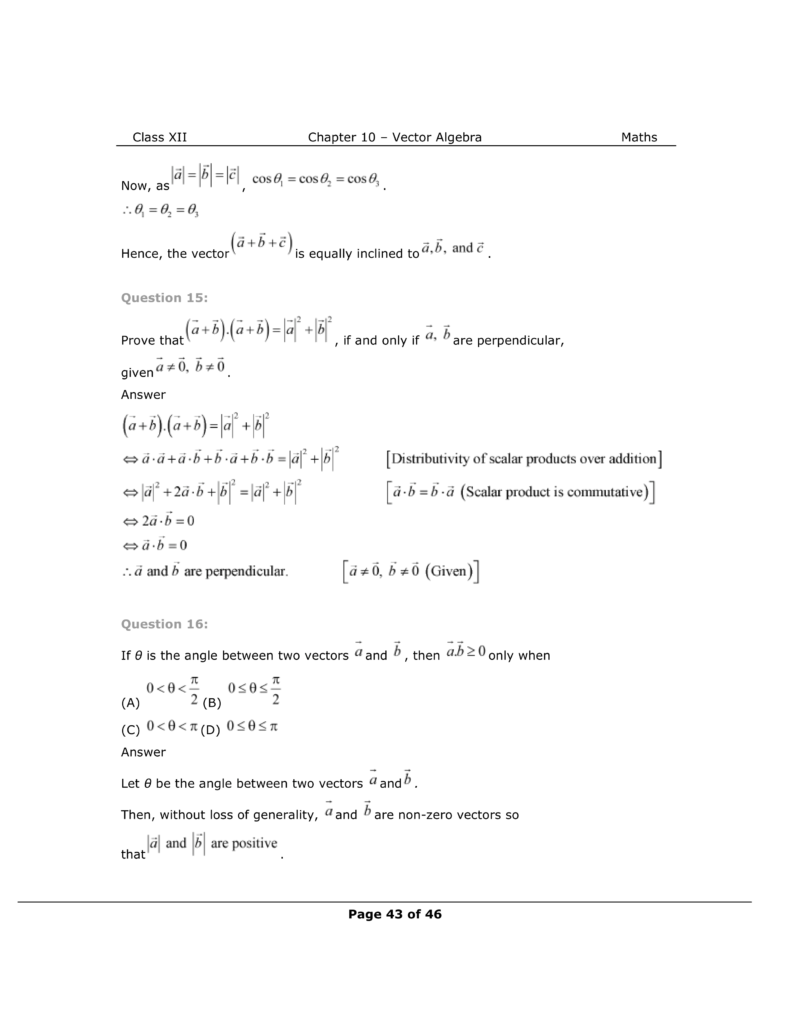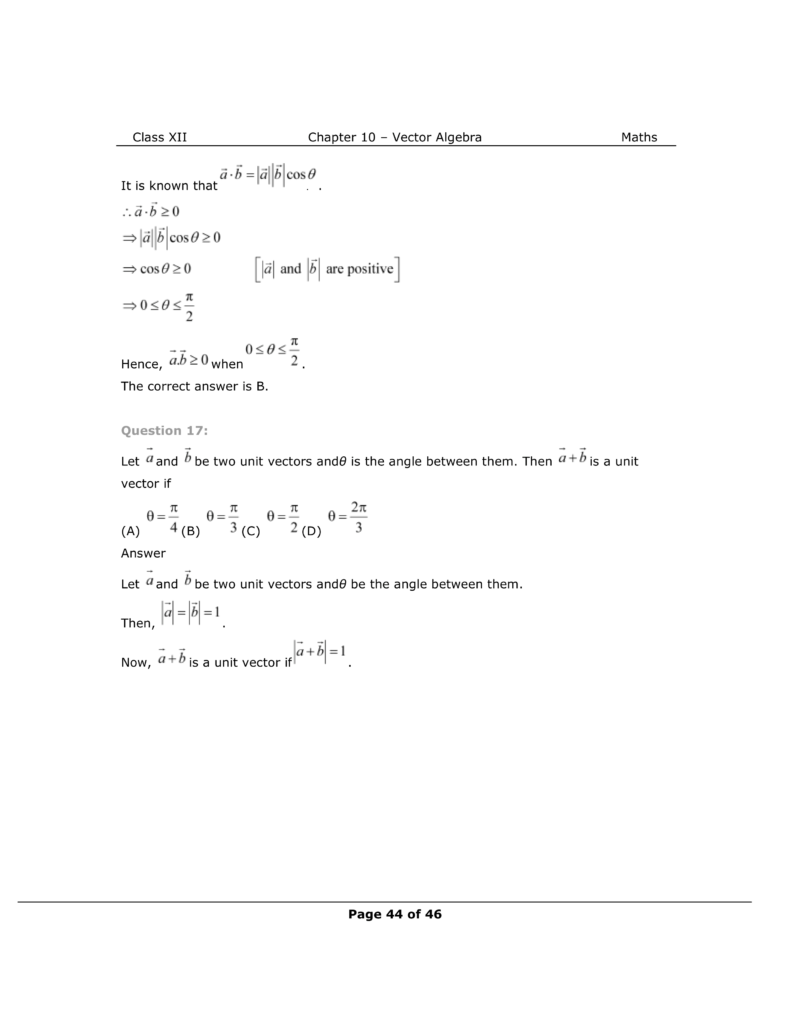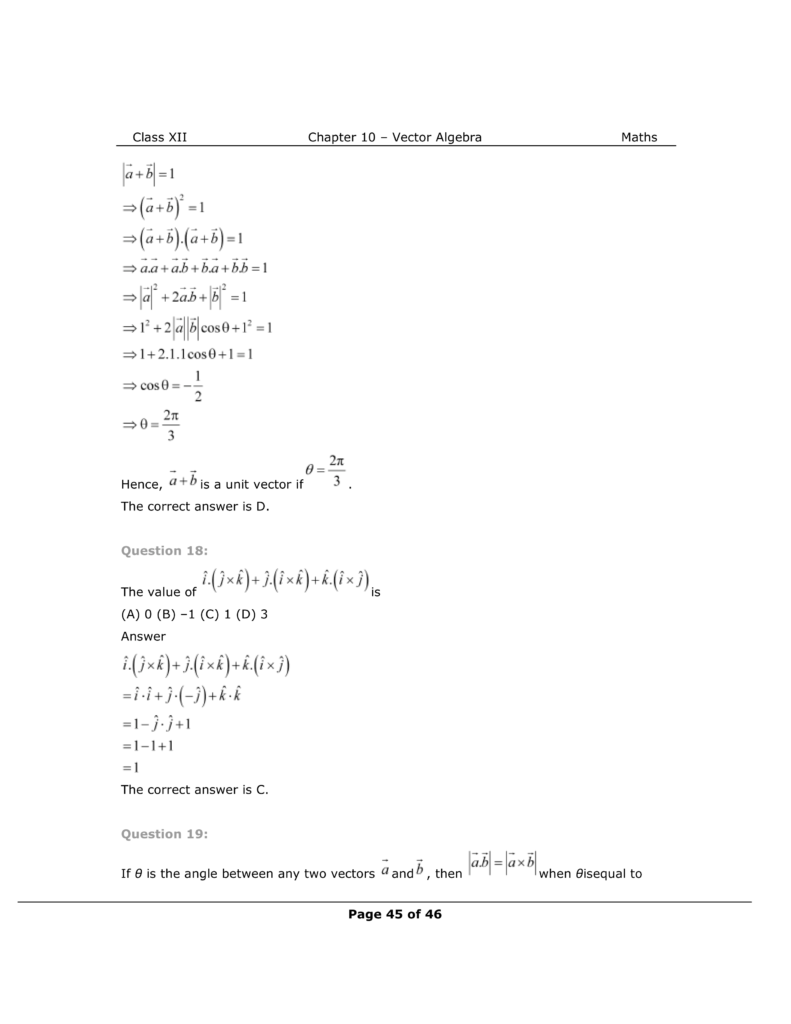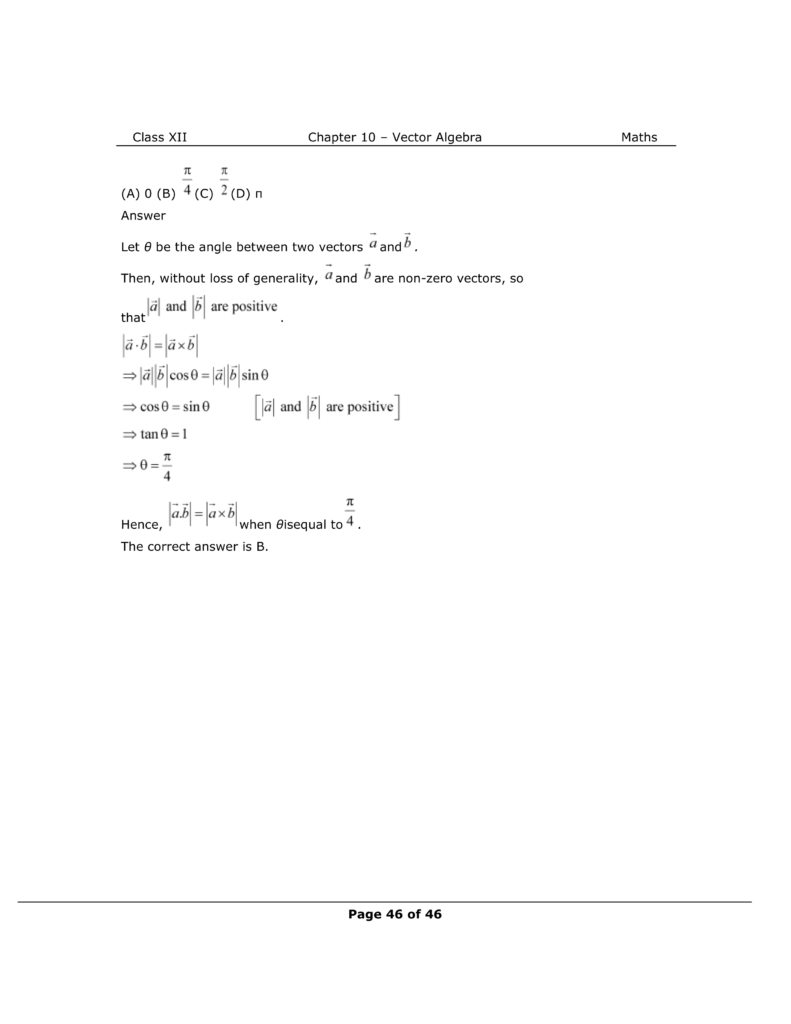NCERT Class 12 Math Book

NCERT Class 12 Maths Exemplar

Complete Solutions for Class 12 Maths chapter 10

Class 12 maths chapter 9 exercise 9.1 Solutions

Class 12 maths chapter 9 exercise 9.2 Solutions

Class 12 maths chapter 9 exercise 9.3 Solutions

Class 12 maths chapter 9 exercise 9.4 Solutions

Class 12 maths chapter 9 exercise 9.5 Solutions

Class 12 maths chapter 9 exercise 9.6 Solutions

Class 12 maths chapter 9 Miscellaneous exercise Solutions

If you have any Confusion related to NCERT Solutions For Class 12 Maths Chapter 10 Miscellaneous Exercise then feel free to ask in the comments section down below.

To watch Free Learning Videos on Class 12 by Kota’s top Faculties Install the eSaral App